﻿ 纵向数据下均值协方差模型的贝叶斯统计诊断

纵向数据下均值协方差模型的贝叶斯统计诊断Bayesian Statistical Diagnosis of Joint Mean and Covariance Models with Longitudinal Data

Abstract: Bayesian statistical diagnosis of joint mean and covariance models with longitudinal data is studied. By combining the Gibbs sampler and Metropolis-Hastings algorithm, the Bayesian case deletion diagnosis statistic is obtained to identify data outliers. Simulation study and a real data analysis show that the proposed diagnosis method is feasible and effective.

1. 引言

2. 模型与符号

2.1. 纵向数据下均值协方差模型

${\mu }_{ij}={X}_{ij}^{\text{T}}\beta ,\text{\hspace{0.17em}}{l}_{ijk}={Z}_{ijk}^{\text{T}}\gamma ,\text{\hspace{0.17em}}\mathrm{log}\left({\sigma }_{ij}^{2}\right)={H}_{ij}^{\text{T}}\lambda$ (1)(2)

2.2. K-L距离

K-L距离也叫K-L信息，具有距离和信息的某些性质，在统计上以反映两个模型或分布的差异而著

3. 贝叶斯统计诊断

3.1. 先验分布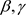相互独立且具有正态先验分布，分别为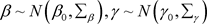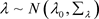，其中假设超参数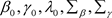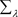是已知的。

3.2. Gibbs抽样和条件分布

· 抽样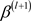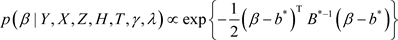, (3)

· 抽样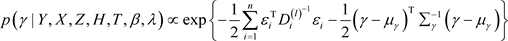(4)

· 抽样(5)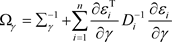,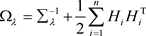.

3.3. 贝叶斯估计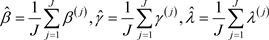3.4. 贝叶斯数据删除影响诊断, (6)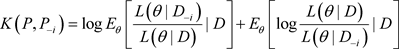, (7)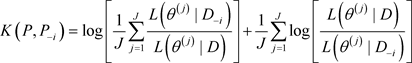. (8)

4. 模拟研究

TypeI：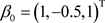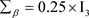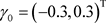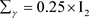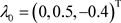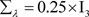。这种设置具有很好的先验信息。

TypeII：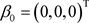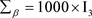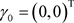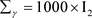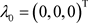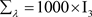。这些超参数值的设置代表的是没有先验信息的情况。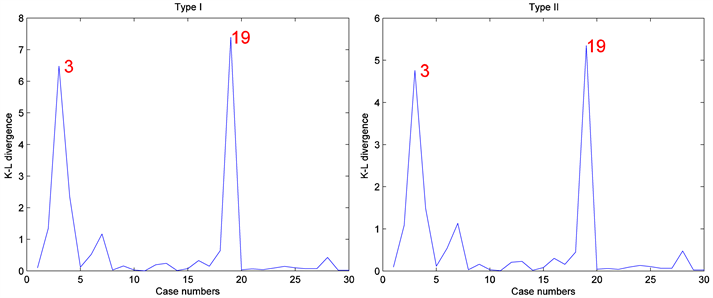Figure 1. Results based on Bayesian case deletion diagnosis when n = 30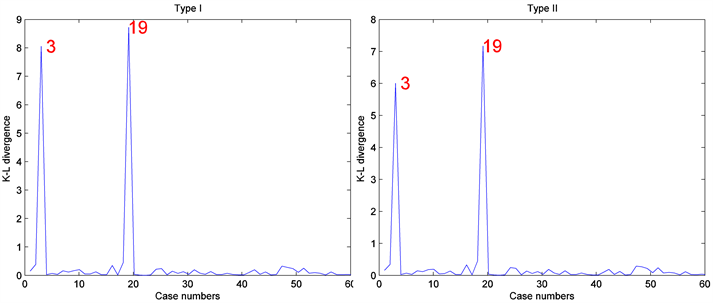Figure 2. Results based on Bayesian case deletion diagnosis when n = 60

5. 实例分析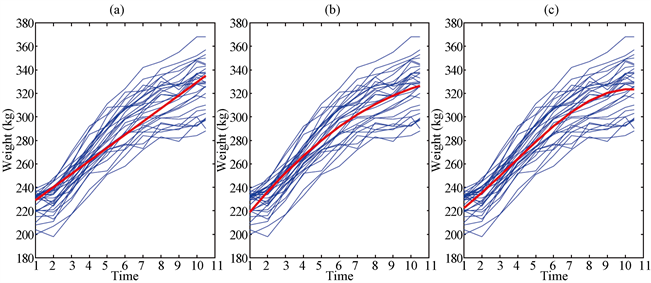Figure 3. Plot for the cattle data and the thicker line is the polynomial fitted curve: (a) linear polynomial fitted curve; (b) quadratic polynomial fitted curve and (c) cubic polynomial fitted curve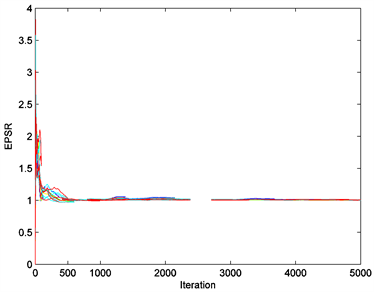Figure 4. EPSR values of all parameters in the cattle data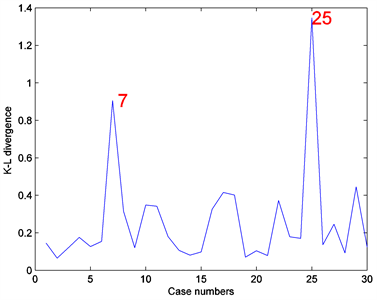Figure 5. Statistical diagnosis results of the cattle data

6. 结论

 Pourahmadi, M. (1999) Joint Mean-Covariance Models with Applications to Longitudinal Data: Unconstrained Parameterization. Biometrika, 86, 677-690.
https://doi.org/10.1093/biomet/86.3.677

 Pourahmadi, M. (2000) Maximum Likelihood Estimation for Generalised Linear Models for Multivariate Normal Covariance Matrix. Biometrika, 87, 425-435.
https://doi.org/10.1093/biomet/87.2.425

 Pan, J.X. and MacKenzie, G. (2003) On Modelling Mean-Covariance Structures in Longitudinal Studies. Biometrika, 90, 239-244.
https://doi.org/10.1093/biomet/90.1.239

 Mao, J. and Zhu, Z.Y. (2011) Joint Semiparametric Mean-Covariance Model in Longitudinal Study. Science China Mathematics, 54, 145-164.
https://doi.org/10.1007/s11425-010-4078-4

 Rothman, A.J., Levina, E. and Zhu, J. (2010) A New Approach to Cholesky-Based Covariance Regularization in High Dimensions. Biometrika, 97, 539-550.
https://doi.org/10.1093/biomet/asq022

 Zhang, W.P. and Leng, C.L. (2012) A Moving Average Cholesky Factor Model in Covariance Modeling for Longitudinal Data. Biometrika, 99, 141-150.
https://doi.org/10.1093/biomet/asr068

 Xu, D.K., Zhang, Z.Z. and Wu, L.C. (2013) Joint Variable Selection of Mean-Covariance Model for Longitudinal Data. Open Journal of Statistics, 3, 27-35.
https://doi.org/10.4236/ojs.2013.31004

 Cook, R.D. (1977) Detection of Influential Observations in Linear Regression. Technometrics, 19, 15-18.
https://doi.org/10.1080/00401706.1977.10489493

 Cho, H., Ibrahim, J.G., Sinha, D. and Zhu, H.T. (2009) Bayesian Case Influence Diagnostics for Survival Models. Biometrics, 65, 116-124.
https://doi.org/10.1111/j.1541-0420.2008.01037.x

 赵远英, 徐登可, 庞一成. 联合均值与方差模型的Bayes分析[J]. 高校应用数学学报, 2018, 33(2): 241-252.

 戴琳, 陶治, 吴刘仓. 联合均值与方差模型的统计诊断[J]. 统计与信息论坛, 2017, 32(1): 14-19.

 Tang, N.S. and Duan, X.D. (2012) A Semiparametric Bayesian Approach to Generalized Partial Linear Mixed Models for Longitudinal Data. Computational Statistics and Data Analysis, 56, 4348-4365.
https://doi.org/10.1016/j.csda.2012.03.018

 Ye, H.J. and Pan, J.X. (2006) Modelling of Covariance Structures in Generalized Estimating Equations for Longitudinal Data. Biometrika, 93, 927-941.
https://doi.org/10.1093/biomet/93.4.927

 韦博成. 参数统计教程[M]. 北京: 高等教育出版社, 2006.

 Geyer, C.J. (1992) Practical Markov Chain Monte Carlo. Statistical Science, 7, 473-511.
https://doi.org/10.1214/ss/1177011137

 Kenward, M.G. (1987) A Method for Comparing Profiles of Repeated Measurements. Applied Statistics, 36, 296-308.
https://doi.org/10.2307/2347788

Top